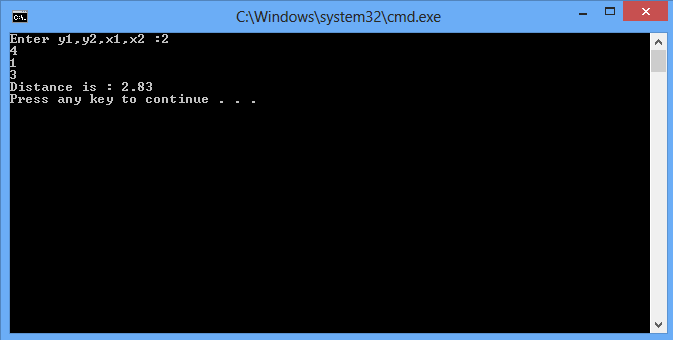# Introduction to Pointers

In this part you will learn: 1. What are Pointers 2. How to use them in Programs 3. C syntax 4. Showing output What are Pointers? Our computer stores variables in the memory so they can be accessed by the compiler for processsing. The compiler sets aside a memory location with a unique address to store that variable. Compiler basically creates an address space for a specific variable declared in the program and pointers are used to store the address locations of the variables or pointer can be referred as a variable that stores a memory address. A pointer variable can be declared just by putting an asterisk (*) before the name of the variable but after the type of variable e.g if we want to make an integer type pointer variable then simply put asterisk before the name of the variable like here we are making an integer type pointer named num1 so we write it as int *num1; Secondly there is an important thing that you will see in the program below that is ampersand ‘&’ , whenever we want that the change from the function changes the value of originally passed variable , we used ampersand sign with the variable when being passed. The address of the variable is passed when we write ampersand with variable and ampersand is opposite to *. e.g int num1=4; printf(“Address of num1 : %d”,&num1); // here something like 00AF344 will show up which is the //memory address now if we want to see the relationship between ampersand and pointers it can be demonstrated as printf(“Value of num1 : %d”,&*num1); // output will be value of num1 i.e 4 Pointer dereferences the variable. Using Pointer and Functions Basic Step: Open Dev C++ then File > new > source file and start writing the code below.
`#include<stdio.h>#include<conio.h>#include<math.h>`
These are the header files that we need for coding. The main header is stdio.h which provides us with most of the functions for coding in C and the second header files is just for using a function which can pause the screen until the user hits any key.
`double distance(int *,int *,int *,int *); int main(){	int y1,y2,x1,x2;	printf("Enter y1,y2,x1,x2 :");	scanf("%d%d%d%d",&y1,&y2,&x1,&x2); 	printf("Distance is : %.2lf \n",distance(&y1,&y2,&x1,&x2)); }`
In the first part of the code we have declared a function that will take in 4 pointer variables as indicated. These four variables are basically the 2D coordinates of 2 points between which the distance will be calculated by the distance function. In the main part we have declared four integer type variables and have taken their values by the user . The variables are passed into the functions with ampersand(&) because when they are later used in the function that is having pointer variables as its input , the variables get the values as input by the user. Whenever the pointers are used in a function the values through main are always passed by reference.
`	double distance(int *y1,int *y2,int *x1,int *x2){	double x,y;	x = *x2-*x1;	y = *y2-*y1;	 return sqrt(pow(y,2) + pow(x,2));}`
In this function , the inputs are four pointer type integer type variables. Passed from main are the variables with ampersand sign. Here we used pointer variables to perform the operation of addition and subtraction instead of using the normal variables because we want to use user’s provided input values. Below we see function sqrt(pow(y,2) + pow(x,2)), this is a built in C function which comes from math.h library , pow function simply takes power between two numbers and it takes a variables as it first input and the power to which we want the value of the variable to be raised as second input. Sqrt function simply takes the square root of its input. This function will return the distance between the two numbers by using the distance formula. The returned number will have double variable type. Execute > compile then Execute > run Output